### 機器學習回顧篇（4）：邏輯回歸

• 2019 年 10 月 3 日
• 筆記

# 2 邏輯回歸原理

## 2.1 從線性回歸到邏輯回歸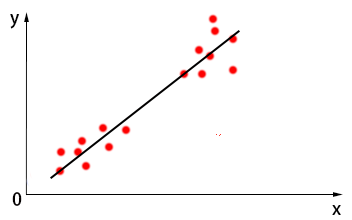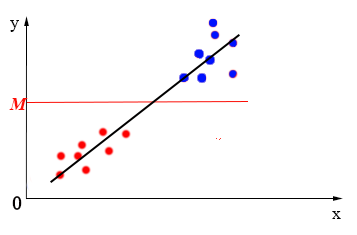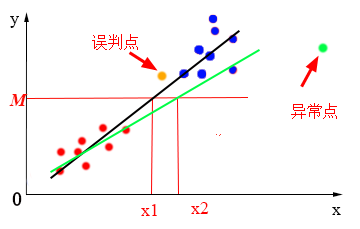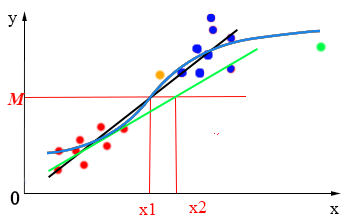## 2.2 sigmod函數

sigmoid函數也叫Logistic函數，函數表達式如下：

\$g(z)=frac{1}{1+{{e}^{-x}}}\$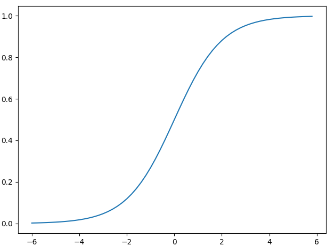[y=left{ _{0,text{    }g(x)<0.5}^{1,text{    }g(x)ge 0.5} right.]

[g(x)=frac{1}{1+{{e}^{-z}}}]

[z=f(x)={{theta }_{0}}+{{theta }_{1}}{{x}_{1}}+{{theta }_{2}}{{x}_{2}}+cdots +{{theta }_{n}}{{x}_{n}}]

[h(x)=frac{1}{1+{{e}^{-({{theta }_{0}}+{{theta }_{1}}{{x}_{1}}+{{theta }_{2}}{{x}_{2}}+cdots +{{theta }_{n}}{{x}_{n}})}}}]

[h(x)=g(z)=g({{theta }^{T}}x)=frac{1}{1+{{e}^{-{{theta }^{T}}x}}}]

{{theta }_{0}}  \
{{theta }_{1}}  \
vdots   \
{{theta }_{n}}  \
end{matrix} right]\$，\$x=left[ begin{matrix}
{{x}_{0}}  \
{{x}_{1}}  \
vdots   \
{{x}_{n}}  \
end{matrix} right]\$

# 3 損失函數

[p(y=1|x;theta )=h(x)]

[p(y=0|x;theta )=1-h(x)]

[cos t(h(x),y)=left{ _{-log (1-h(x)),text{ }y=0}^{-log (h(x)),text{ }y=1} right.]

[J(theta )=-frac{1}{m}sumlimits_{i=1}^{m}{cos (h(x),y)}]

[J(theta )=-frac{1}{m}sumlimits_{i=1}^{m}{({{y}_{i}}log (h({{x}_{i}}))+(1-{{y}_{i}})log (1-h({{x}_{i}})))}]

# 4 代碼實現

`import torch  from torch import nn  from torch.autograd import Variable  import matplotlib.pyplot as plt  import numpy as np    # 假數據  n_data = torch.ones(100, 2)         # 數據的基本形態  x0 = torch.normal(2*n_data, 1)      # 類型0 x data (tensor), shape=(100, 2)  y0 = torch.zeros(100)               # 類型0 y data (tensor), shape=(100, 1)  x1 = torch.normal(-2*n_data, 1)     # 類型1 x data (tensor), shape=(100, 1)  y1 = torch.ones(100)                # 類型1 y data (tensor), shape=(100, 1)    # 注意 x, y 數據的數據形式是一定要像下面一樣 (torch.cat 是在合併數據)  x = torch.cat((x0, x1), 0).type(torch.FloatTensor)  # FloatTensor = 32-bit floating  y = torch.cat((y0, y1), 0).type(torch.FloatTensor)    # LongTensor = 64-bit integer    # 畫圖  # plt.scatter(x.data.numpy()[:, 0], x.data.numpy()[:, 1], c=y.data.numpy(), s=100, lw=0, cmap='RdYlGn')  # plt.show()    class LogisticRegression(nn.Module):      def __init__(self):          super(LogisticRegression, self).__init__()          self.lr = nn.Linear(2, 1)          self.sm = nn.Sigmoid()        def forward(self, x):          x = self.lr(x)          x = self.sm(x)          return x    logistic_model = LogisticRegression()  if torch.cuda.is_available():      logistic_model.cuda()    # 定義損失函數和優化器  criterion = nn.BCELoss()  optimizer = torch.optim.SGD(logistic_model.parameters(), lr=1e-3, momentum=0.9)    # 開始訓練  for epoch in range(10000):      if torch.cuda.is_available():          x_data = Variable(x).cuda()          y_data = Variable(y).cuda()      else:          x_data = Variable(x)          y_data = Variable(y)        out = logistic_model(x_data)      loss = criterion(out, y_data)      print_loss = loss.data.item()      mask = out.ge(0.5).float()  # 以0.5為閾值進行分類      correct = (mask == y_data).sum()  # 計算正確預測的樣本個數      acc = correct.item() / x_data.size(0)  # 計算精度      optimizer.zero_grad()      loss.backward()      optimizer.step()      # 每隔20輪打印一下當前的誤差和精度      if (epoch + 1) % 20 == 0:          print('*'*10)          print('epoch {}'.format(epoch+1)) # 訓練輪數          print('loss is {:.4f}'.format(print_loss))  # 誤差          print('acc is {:.4f}'.format(acc))  # 精度    # 結果可視化  w0, w1 = logistic_model.lr.weight  w0 = float(w0.item())  w1 = float(w1.item())  b = float(logistic_model.lr.bias.item())  plot_x = np.arange(-7, 7, 0.1)  plot_y = (-w0 * plot_x - b) / w1  plt.scatter(x.data.numpy()[:, 0], x.data.numpy()[:, 1], c=y.data.numpy(), s=100, lw=0, cmap='RdYlGn')  plt.plot(plot_x, plot_y)  plt.show()`

# 4 總結

1）預測結果是介於0和1之間的概率；

2）可以適用於連續性和類別性自變量；

3）容易使用和解釋。

1）對模型中自變量多重共線性較為敏感，例如兩個高度相關自變量同時放入模型，可能導致較弱的一個自變量回歸符號不符合預期，符號被扭轉。需要利用因子分析或者變量聚類分析等手段來選擇代表性的自變量，以減少候選變量之間的相關性；

2）預測結果呈“S”型，因此從log(odds)向概率轉化的過程是非線性的，在兩端隨着log(odds)值的變化，概率變化很小，邊際值太小，slope太小，而中間概率的變化很大，很敏感。 導致很多區間的變量變化對目標概率的影響沒有區分度，無法確定閥值。

https://blog.csdn.net/out_of_memory_error/article/details/81275651

https://www.cnblogs.com/yiduobaozhiblog1/p/8872903.html

https://blog.csdn.net/ligang_csdn/article/details/53838743

https://baijiahao.baidu.com/s?id=1620514366177013756&wfr=spider&for=pc